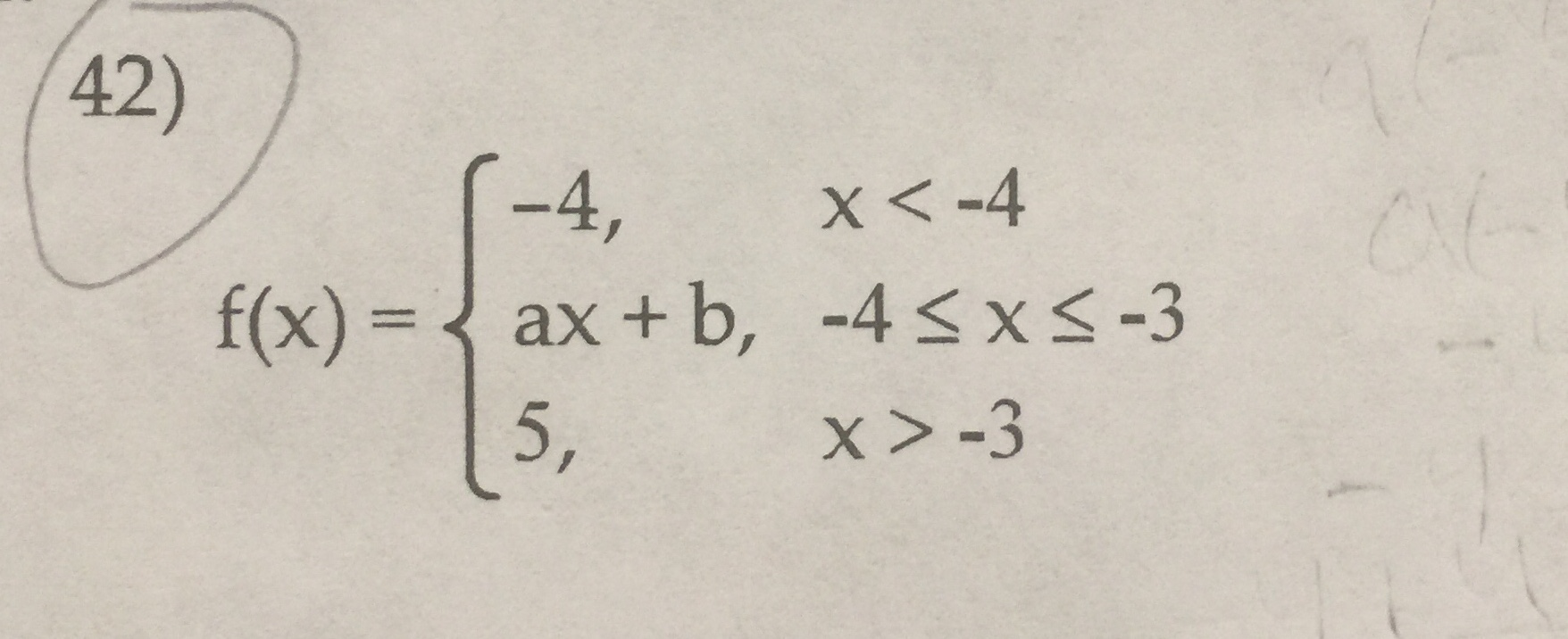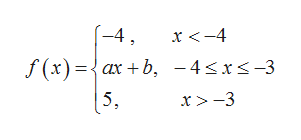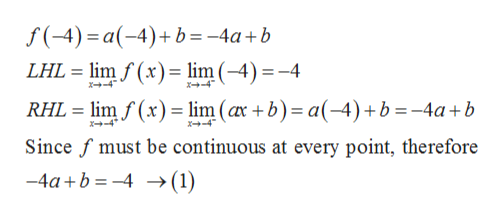42)(-4,X-4ax + b, -4s x< -3f(x)=5,x > -3

Question

Find numbers a and b so that f is continuous at every point.help_outlineImage Transcriptionclose42) (-4, X-4 ax + b, -4s x< -3 f(x)= 5, x > -3 fullscreen
Step 1

Given,help_outlineImage Transcriptionclose(-4 x<-4 f (x)={ax + b, -4sxs-3 5, x > -3 fullscreen
Step 2

We check the continuity of the given function at the points x = -4 and x = -3.

Step 3

At x = -4:

&nb...help_outlineImage Transcriptionclosef(-4) a-4)b=-4a+b LHL lim f(x) = lim (-4) =-4 x+-4 X-4 RHL limf(x)lim (ax +b) a-4) +b = -4a+b *-4 x+-4 Since f must be continuous at every point, therefore -4ab4 >(1) fullscreen

Want to see the full answer?

See Solution

Want to see this answer and more?

Our solutions are written by experts, many with advanced degrees, and available 24/7

See Solution
Tagged in

Calculus